### Home > A2C > Chapter 10 > Lesson 10.2.6 > Problem10-156

10-156.
1. Simplify each expression. Homework Help ✎

1.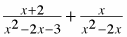2.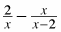3.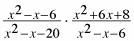4.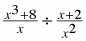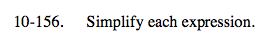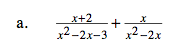First, simplify the second fraction.

$\frac{1}{\textit{x}-2}$

Now multiply to equalize the denominators.

$\frac{\textit{x}^{2}-4}{(\textit{x}-3)(\textit{x}+1)(\textit{x}-2)}+\frac{\textit{x}^{2}-2\textit{x}-3}{(\textit{x}-3)(\textit{x}+1)(\textit{x}-2)}$

$\frac{2x^{2}-2\textit{x}-7}{(\textit{x}-3)(\textit{x}+1)(\textit{x}-2}$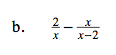See part (a).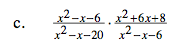Eliminate x2x − 6.

$\frac{x^{2}+6x+8}{x^{2}-x-20}$

Factor each polynomial.

$\frac{(x+2)(x+4)}{(x-5)(x+4)}$

$\frac{x+2}{x-5}$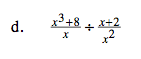Remember to flip the second fraction, then multiply.

See part (c).